# Completing The Square Worksheet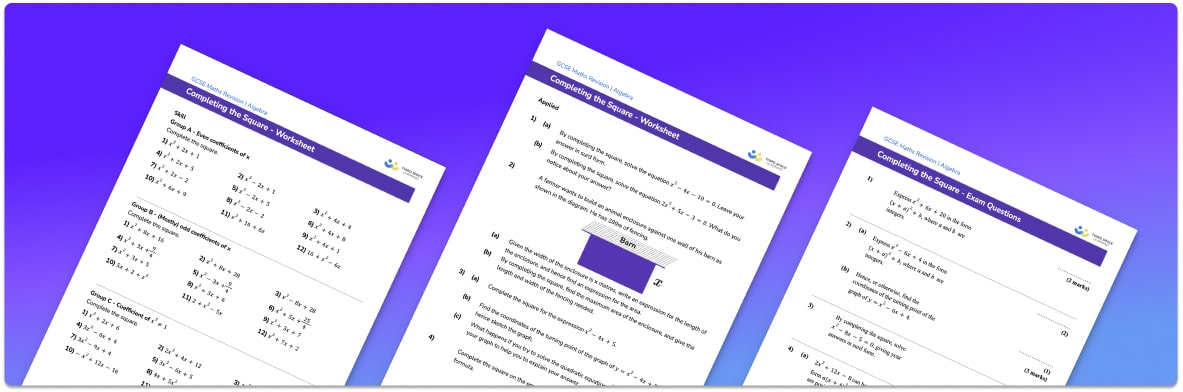• This field is for validation purposes and should be left unchanged.

You can unsubscribe at any time (each email we send will contain an easy way to unsubscribe). To find out more about how we use your data, see our privacy policy.

• Section 1 of the completing the square worksheet contains 36 skills-based questions, in 3 groups to support differentiation
• Section 2 contains 4 applied completing the square questions with a mix of worded problems and deeper problem solving questions
• Section 3 contains 5 higher level GCSE exam questions to help with the practice of completing the square
• Answers and a mark scheme for all questions are provided
• Questions follow variation theory with plenty of opportunities for students to work independently at their own level
• All questions created by fully qualified expert secondary maths teachers
• Suitable for GCSE maths revision for AQA, OCR and Edexcel exam boards

### Completing the square at a glance

Completing the square involves rearranging quadratic expressions into a perfect square form. We can use completing the square as a method for solving quadratic equations by rearranging the equation so that the perfect square is on one side of the equation, and all other terms are on the other side. We then need to isolate the unknown variable by square rooting both sides of the equation, remembering that a square root has a positive and a negative answer. A strong understanding of the order of operations is very important.

The solutions of the quadratic equations may be left as surds or written as decimals as required.

Completing the square can also be linked to transformation of curves and the graphs of quadratic functions. A quadratic function written in its completing the square form gives the turning point of its parabola which can be used to help to sketch quadratic graphs. Completing the square can also be used to show how the quadratic formula is formed with a, b and c as the coefficients of the terms.

Looking forward, students can then progress to additional algebra worksheets, for example a factorising worksheet, or a simultaneous equations worksheet.For more teaching and learning support on Algebra our GCSE maths lessons provide step by step support for all GCSE maths concepts.

## Related worksheets

Solving Equations with Fractions Worksheet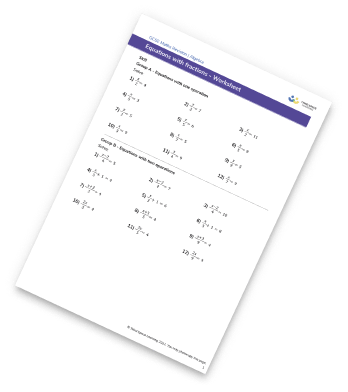Linear Equations Worksheet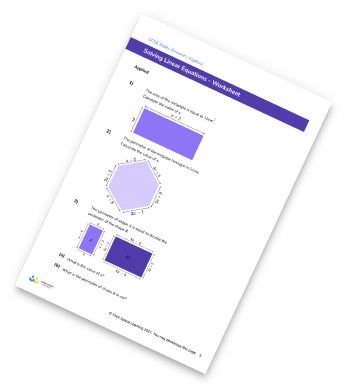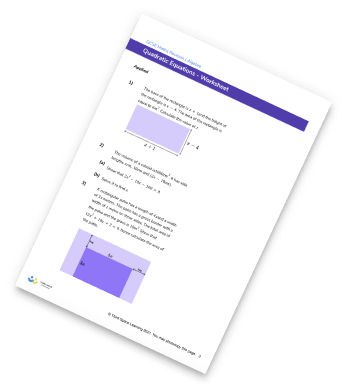Solving Equations Worksheet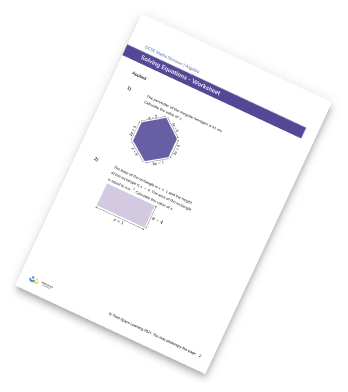## Do you have KS4 students who need more focused attention to succeed at GCSE?There will be students in your class who require individual attention to help them succeed in their maths GCSEs. In a class of 30, it’s not always easy to provide.

Help your students feel confident with exam-style questions and the strategies they’ll need to answer them correctly with our dedicated GCSE maths revision programme.

Lessons are selected to provide support where each student needs it most, and specially-trained GCSE maths tutors adapt the pitch and pace of each lesson. This ensures a personalised revision programme that raises grades and boosts confidence.

Find out more